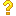•闪存
•博客
• 发言小组
• 投递新闻
• 提问博问
• 添加收藏
• 发布招聘
•文库

8

##10这个一段js脚本 执行后 是一个数字 哪位高手知道它是怎么计算的

<script>___=(____=-~(___=-~(__=-~-~[])))+-~-~-~-~____*(-~[]<<(__))+(-~[]<<(___))*(-~[]<<(___))+(-~[]
5